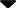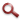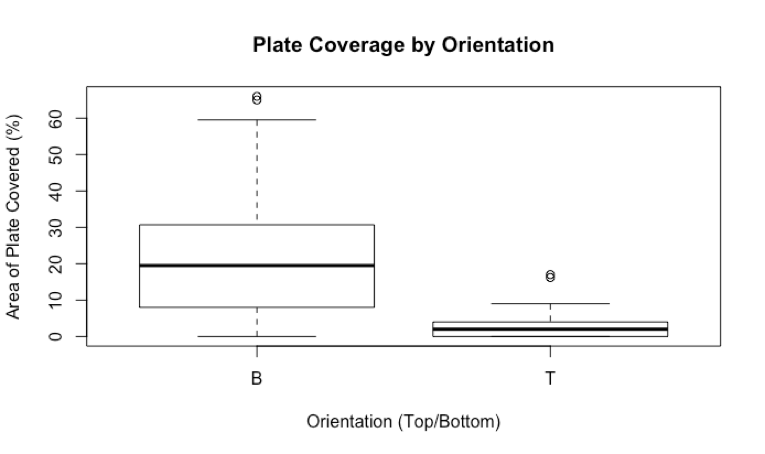Site WebYou are here:

## Student Project FiguresFigure 10 Figure 10: Comparative boxplots of the percentage area of ARMS plate covered by Didemnum on the top (mean = 2.99%, sd=3.82, n=53) and bottom (mean = 21.79%, sd=17.29, n=53), sides. Difference between means was significant, p<<0.001 (t=7.7257, df=57.05 at 95% CI). Figure 10